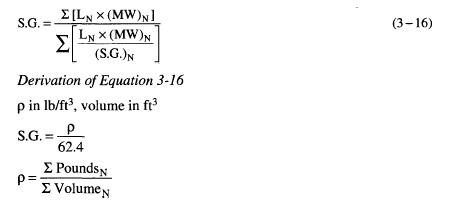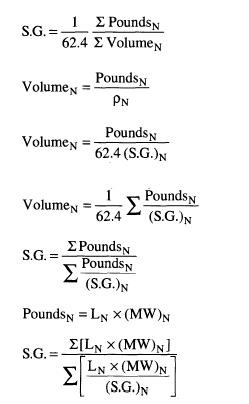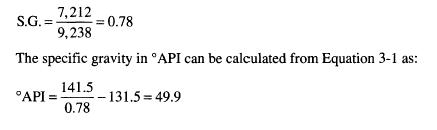# Specific Gravity of Liquid

Remembering that the weight of each component is the number of moles of that component times its molecular weight (pounds = MW X moles), the specific gravity of the liquid is given by:Column 5 lists a specific gravity for each component in the liquid phase at standard conditions except as noted. It would be more accurate to adjust these gravities for the actual pressure and temperature of the fluid being flashed. This will have a marginal effect on the results as the characterization of the heptanes is of overriding importance to the calculation. If this is not known for the pressure and temperature of the flash it can be approximated from known conditions using Figure 3-8.

Column 6 is derived by dividing Column 4 by Column 5. The liquid specific gravity is: